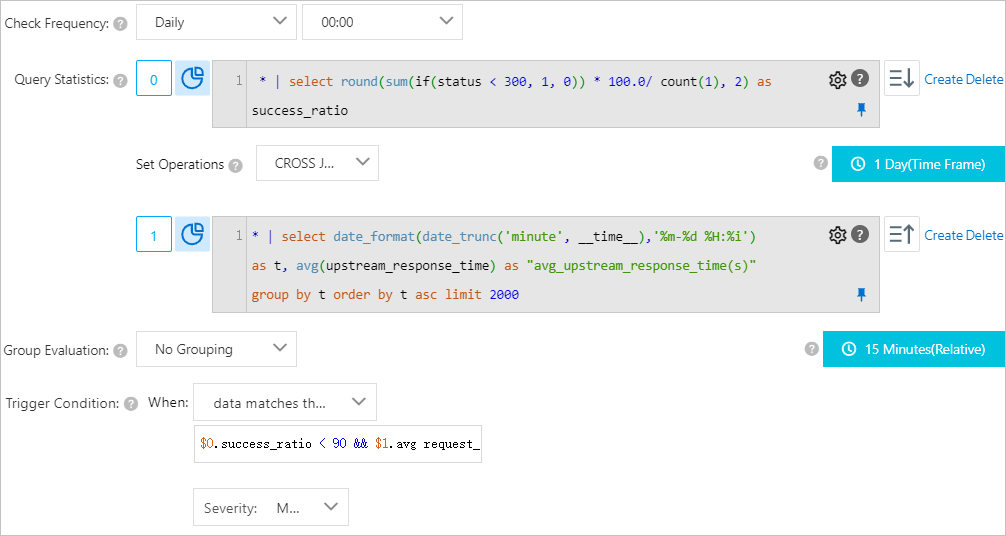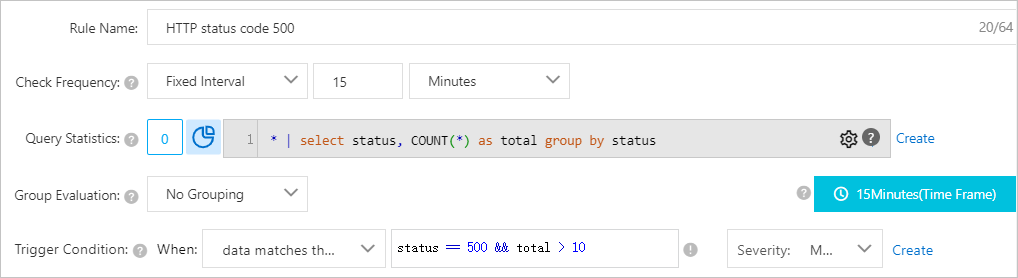In an alert monitoring rule, the execution result of an evaluate expression is used to check whether the specified trigger condition is met. The execution result of an evaluate expression is also used to dynamically evaluate the severity of an alert. The result of a specified query statement is an input, and the fields in the result of set operations are variables. If the condition that is specified in an evaluate expression is true, and matches the specified threshold of continuous triggers, an alert is triggered. This topic describes how to use evaluation expressions.

Limits

The evaluate expressions that can be specified in an alert monitoring rule have the following limits:
• Negative numbers must be enclosed in parentheses (), for example, x+(-100)<100.
• Numeric values are converted into 64-bit floating-point numbers. If a comparison is used, errors may occur.
• Variable names can only contain letters and digits, and must start with a letter.
• An evaluate expression must be 1 to 128 characters in length.
• An alert is triggered only when the result of the specified evaluate expression is true and matches the specified threshold of continuous triggers. For example, an evaluate expression is 100+100. In this case, the result is 200 and is not true, no alert is triggered.
• Log Service reserves the two words: true and false. It also reserves two special characters: dollar sign (\$) and period (.).These words or characters cannot be used as variables.

Syntax

The following syntax types are supported for the evaluate expressions of an alert monitoring rule.
Syntax type Description Example
Arithmetic operators The addition (+), subtraction (-), multiplication (*), division (/), and modulus (%) operators are supported.

+-*/%

• x * 100 + y > 200
• x % 10 > 5
Comparison operators The following eight comparison operators are supported: greater-than (>), greater-than-or-equal-to (>=), less-than (<), less-than-or-equal-to (<=), equal-to (==), not-equal-to (!=), regex match (=~), and regex not match (!~).
Note
• Backslashes (\) in regular expressions must be escaped.
• The Regular Expression 2 (RE2) syntax is used. For more information, see Syntax.
• x >= 0
• x < 100
• x <= 100
• x == 100
• x == "foo"
• Regex match: x =~ "\\w +"
Logical operators The AND (&&) and OR (||) operators are supported.
• x >= 0 && y <= 100
• x > 0 || y >0
Not operator The not operator (!) is supported. !(a < 1 && a > 100)
Numeric constants Numeric constants are supported. Log Service converts numeric constants into 64-bit floating-point numbers. x > 100
String constants String constants are supported. The string constants are in the format of 'String', for example, 'string'. foo == 'String'
Boolean constants Boolean constants are supported. Valid values: true and false. (x > 100) == true
Parentheses Parentheses () can be used to override the standard precedence order and force Log Service to evaluate the enclosed part of a trigger condition before an unenclosed part. x * (y + 100) > 100
contains function The contains function can be used to check whether a string contains a substring. For example, if you invoke contains(foo, 'hello'), and true is returned, this indicates that the foo string contains the hello substring. contains(foo, 'hello')

Evaluate the results of set operations

Log Service supports associated monitoring and evaluates the results of set operations for up to three sets. For more information, see Multi-set operations.

You can use variables in an evaluate expression. For more information, see Use an evaluate expression to specify a trigger condition.

Operation methods

Note
• Log Service converts all numeric values into 64-bit floating-point numbers.
• A string constant must be enclosed in single quotation marks ('') or double quotation marks (""), for example, 'String' or "String".
• Boolean values include true and false.
Operator Operation method
Operation between variables Operation between a non-string constant and a variable Operation between a string constant and a variable
Arithmetic operators: addition (+), subtraction (-), multiplication (*), division (/), and modulus (%) Before an arithmetic operator is applied, the left and right operands are converted into 64-bit floating-point numbers. Before an arithmetic operator is applied, the left and right operands are converted into 64-bit floating-point numbers. Unsupported.
Comparison operators:

greater-than (>), greater-than-or-equal-to (>=), less-than (<), less-than-or-equal-to (<=), equal-to (==), and not-equal-to (!=)

Log Service uses the following comparison rules that are sorted in the precedence order:
1. The left and right operands are converted into 64-bit floating-point numbers and then compared based on the numerical order. If the conversion fails, the operation of the next priority is performed.
2. The left and right operands are converted into strings and then compared based on the lexicographic order.
The left and right operands are converted into 64-bit floating-point numbers and then compared based on the numerical order. The left and right operands are converted into strings and then compared based on the lexicographic order.
Matching operators:

regex match (=~) and regex not match (!~)

Before a matching operator is applied, the left and right operands are converted into strings. Unsupported. Before a matching operator is applied, the left and right operands are converted into strings.
Logical operators:

AND (&&) and OR (||)

The left and right operands must be sub-expressions and the result of the operation must be a Boolean value. For example, the evaluate expression is \$0.success_ratio < 90 && \$1.average_upstream_response_time(s) > 60. The left and right operands must be sub-expressions and the result of the operation must be a Boolean value. For example, the evaluate expression is \$0.success_ratio < 90 && \$1.average_upstream_response_time(s) > 60. The left and right operands must be sub-expressions and the result of the operation must be a Boolean value. For example, the evaluate expression is \$0.success_ratio < 90 && \$1.average_upstream_response_time(s) > 60.
Not operator (!) The specified operand must be a sub-expression and the result of the operation must be a Boolean value. For example, the evaluate expression is !(\$0.success_ratio < 90).

This operator is not supported for the fields in the result of set operations.

The specified operand must be a sub-expression and the result of the operation must be a Boolean value. For example, the evaluate expression is !(\$0.success_ratio < 90).

This operator is not supported for the fields in the result of set operations.

The specified operand must be a sub-expression and the result of the operation must be a Boolean value. For example, the evaluate expression is !(\$0.success_ratio < 90).

This operator is not supported for the fields in the result of set operations.

contains function Before the contains function is run, the left and right operands are converted into strings. Unsupported. Before the contains function is run, the left and right operands are converted into strings.
Parentheses () Parentheses () are used to override the standard precedence order and force Log Service to evaluate the enclosed part of a trigger condition before an unenclosed part. Parentheses () are used to override the standard precedence order and force Log Service to evaluate the enclosed part of a trigger condition before an unenclosed part. Parentheses () are used to override the standard precedence order and force Log Service to evaluate the enclosed part of a trigger condition before an unenclosed part.

Examples

• Example 1: If the request success rate is lower than 90% and the response time is higher than 60 seconds within 15 minutes (relative), an alert is generated. The evaluate expression is \$0.success_ratio < 90 && \$1.avg request_time\(s\) > 60. The following figure shows the required settings.• Example 2: If the number of HTTP status code 500 responses within 15 minutes exceeds 10, an alert is triggered. The evaluate expression is status == 500 && total > 10. The following figure shows the required settings.